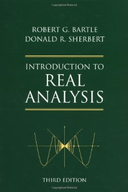Introduction To Real Analysis - 3 Edition - Chapter 2.4 - Problem 11
Register Now

Join StudySoup

Get Full Access to Introduction To Real Analysis - 3 Edition - Chapter 2.4 - Problem 119780471321484

# LetXand Y be nonemptysetsandleth : X x Y --+JRhaveboundedrangeinR Let F: X --+ JR andG

Introduction to Real Analysis | 3rd Edition

Problem 11

LetXand Y be nonemptysetsandleth : X x Y --+JRhaveboundedrangeinR Let F: X --+ JR andG : Y --+ JRbe definedby F(x) := sup{h(x, y) : y e Y}, G(y) := sup{h(x, y) : x eX}. Establish the Principle of the Iterated Suprema: sup{h(x, y) : x eX, y e Y} = sup{F(x) : x eX} = sup{G(y) : y e Y} We sometimes express this in symbols by suph(x, y) = supsuph(x, y) = supsuph(x, y). x,y x y y x 1

Accepted Solution
Step-by-Step Solution:
Step 1 of 3

Advertising Test 3 Study Guide: Key Terms: Chapter 12: Media Planning Essentials • Deal proneness: the ease with which a consumer can get a deal, know what a good deal is, operate with knowledge of what a good price would be, and know a seller’s cost • Price/cost transparency: ease with which consumers can find out the price of a product and the seller’s cost • Above-the-line promotion: traditional measured media advertising; any message broadcast to the public through conventional means such as TV, internet, radio, and magazines • Measured media: media that are closely measured to determine advertising costs and effectiveness; TV, radio, newspaper, magazines and outdoor media • Belo

###### Chapter 2.4, Problem 11 is Solved

Step 2 of 3

Step 3 of 3

Unlock Textbook Solution